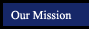Algebra 1 Concepts and Skills
Chapter 5 - Writing Linear Equations
 Section Title Tutorials from this book Examples to Copy (pop-up window) 5.1 Slope-Intercept Form 13 15 17 19 23 25 29 31 37 Finding The Equation in Slope-Intercept Form from a Graph - Practice Game 5.2 Point-Slope Form 15 17 19 21 23 25 27 Finding The Equation of a Line from the Slope and a Point - Practice Game 5.3 Writing Linear Equations Given Two Points 9 11 17 19 25 Finding The Equation of a Line from 2 Points - Practice Game 5.4 Standard Form 15 19 23 29 31 5.5 Modeling with Linear Equations 7 9 13 15 5.6 Perpendicular Lines 11 15 21 27
 Suggested Assignments Section Topic Page Exercises 5.1 Slope-Intercept Form P272-275 x13 – 46 + 3 “examples to copy” 5.2 Point-Slope Form P281-283 x14 – 45 + 3 “examples to copy” 5Q1 Quiz 1 p284 x1 - 15 must show work and check to receive credit 5.3 Writing Linear Equations Given Two Points P288-290 x9 – 36 + 3 “examples to copy” 5.4 Standard Form P294-296 x15 – 44 + 3 “examples to copy” Quiz P297 x1-14 must show work and check to receive credit 5.5 Modeling with Linear Equations P301-304 x6 – 26 + 3 “examples to copy” 5.6 Perpendicular Lines P309-311 x11- 43 + 3 “examples to copy” Chapter Review P313-316 x1-36 show work and check to receive credit Chapter Test P317 x1 - 30

Notes for sections from AlgebraNotes.com

Online Scientific Calculator from Calculator.com

Graphing Calculator by CoolMath.com

Math Glossary (more sophisticated than it looks!)